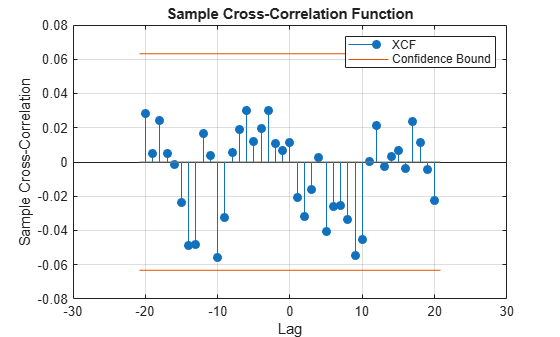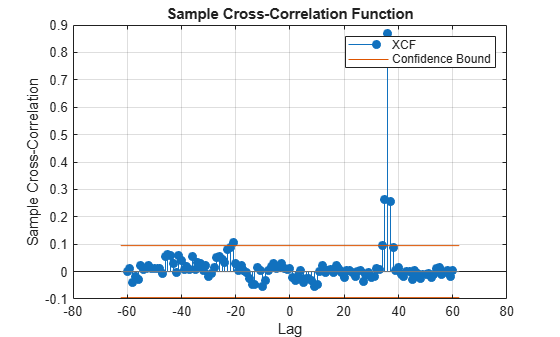# crosscorr

Sample cross-correlation

## Syntax

``crosscorr(y1,y2)``
``crosscorr(y1,y2,Name,Value)``
``xcf = crosscorr(___)``
``````[xcf,lags,bounds] = crosscorr(___)``````
``crosscorr(ax,___)``
``````[xcf,lags,bounds,h] = crosscorr(___)``````

## Description

example

``crosscorr(y1,y2)` plots the cross-correlation function (XCF) between the two univariate, stochastic time series `y1` and `y2` with confidence bounds.`

example

``crosscorr(y1,y2,Name,Value)` uses additional options specified by one or more name-value pair arguments. For example, `crosscorr(y1,y2,'NumLags',10,'NumSTD',2)` plots the sample XCF of `y1` and `y2` for `10` lags and displays confidence bounds consisting of `2` standard errors.`

example

``xcf = crosscorr(___)` returns the sample XCF of `y1` and `y2` using any of the input arguments in the previous syntaxes.`

example

``````[xcf,lags,bounds] = crosscorr(___)``` additionally returns the lag numbers that MATLAB® uses to compute the XCF, and also returns the approximate upper and lower confidence bounds.```
````crosscorr(ax,___)` plots on the axes specified by `ax` instead of the current axes (`gca`). `ax` can precede any of the input argument combinations in the previous syntaxes.```
``````[xcf,lags,bounds,h] = crosscorr(___)``` plots the sample XCF of `y1` and `y2`, and additionally returns handles to plotted graphics objects. Use elements of `h` to modify properties of the plot after you create it.```

## Examples

collapse all

Generate 100 random deviates from a Gaussian distribution with mean 0 and variance 1.

```rng(1); % For reproducibility x = randn(100,1);```

Create a 4-period delayed version of `x`.

`y = lagmatrix(x,4);`

Compute and plot the XCF.

```y(isnan(y)) = 0; % crosscorr does not accept NaNs [XCF,lags,bounds] = crosscorr(x,y); bounds```
```bounds = 2×1 0.2000 -0.2000 ```
`crosscorr(x,y)``bounds` displays the upper and lower confidence bounds, which are the horizontal lines in the XCF plot. As you should expect, `XCF` peaks at lag 4.

Specify the AR(1) model for the first series:

`${y}_{1t}=2+0.3{y}_{1t-1}+{\epsilon }_{t},$`

where ${\epsilon }_{t}$ is Gaussian with mean 0 and variance 1.

`MdlY1 = arima('AR',0.3,'Constant',2,'Variance',1);`

Simulate data from `Mdl`.

```rng(1); T = 1000; y1 = simulate(MdlY1,T);```

Simulate data for the second series by inducing correlation at lag 36.

`y2 = [randn(36,1);y1(1:end-36)+randn(T-36,1)*0.1];`

Plot the XCF using the default settings.

```figure crosscorr(y1,y2,'NumSTD',3)```The plot does not indicate significant cross-correlation between the two series.

Plot the XCF for 60 lags on either side of lag 0.

```figure crosscorr(y1,y2,'NumLags',60,'NumSTD',3)```The plot shows significant correlation at lag 36, as expected.

## Input Arguments

collapse all

First observed univariate time series for which MATLAB estimates or plots the XCF, specified as a numeric vector. The last element of `y1` contains the latest observation.

Data Types: `double`

Second observed univariate time series for which MATLAB estimates or plots the XCF, specified as a numeric vector. The last element of `y2` contains the latest observation.

Data Types: `double`

Axes on which to plot, specified as an `Axes` object.

By default, `crosscorr` plots to the current axes (`gca`).

### Name-Value Pair Arguments

Specify optional comma-separated pairs of `Name,Value` arguments. `Name` is the argument name and `Value` is the corresponding value. `Name` must appear inside quotes. You can specify several name and value pair arguments in any order as `Name1,Value1,...,NameN,ValueN`.

Example: `crosscorr(y1,y2,'NumLags',10,'NumSTD',2)` plots the sample cross correlation between `y1` and `y2` for the lags `-10` to `10` and displays confidence bounds consisting of `2` standard errors.

Number of lags in the sample XCF, specified as the comma-separated pair consisting of `'NumLags'` and a positive integer. `crosscorr` uses lags 0,±1,±2,…,±`NumLags` to estimate the XCF.

The default is `min([20,min(T1,T2) – 1)`, where `T1` and `T2` are the effective sample sizes of `y1` and `y2`.

Example: `crosscorr(y,'NumLags',10)` plots the sample XCF of `y1` and `y2` for lags ` – 10` through `10`.

Data Types: `double`

Number of standard errors in the confidence bounds, specified as the comma-separated pair consisting of `'NumSTD'` and a nonnegative scalar. The confidence bounds are 0 ±`NumSTD*`$\stackrel{^}{\sigma }$, where $\stackrel{^}{\sigma }$ is the estimated standard error of the sample cross-correlation between `y1` and `y2` assuming the series are uncorrelated.

The default yields approximate 95% confidence bounds.

Example: `crosscorr(y,'NumSTD',1.5)` plots the XCF of `y1` and `y2` with confidence bounds `1.5` standard errors away from 0.

Data Types: `double`

## Output Arguments

collapse all

Sample XCF between the univariate time series `y1` and `y2`, returned as a numeric vector of length `2*NumLags` + `1`.

The elements of `xcf` correspond to the elements of `lags`. The center element is the lag 0 cross-correlation.

Lag numbers used for XCF estimation, returned as the numeric vector `(-NumLags):NumLags` having the same orientation as `y1`.

Approximate upper and lower cross-correlation confidence bounds assuming `y1` and `y2` are uncorrelated, returned as a two-element numeric vector.

Handles to plotted graphics objects, returned as a graphics array. `h` contains unique plot identifiers, which you can use to query or modify properties of the plot.

collapse all

### Cross-Correlation Function

The cross-correlation function measures the similarity between a time series and lagged versions of another time series as a function of the lag.

XCF starts with the estimation of the sample cross-covariance function. Consider the time series y1t and y2t and lags k = 0, ±1, ±2,…. For data pairs (y11,y21), (y12,y22),...,(y1T,y2T), an estimate of the lag k cross-covariance is

`${c}_{{y}_{1}{y}_{2}}\left(k\right)=\left\{\begin{array}{c}\frac{1}{T}\sum _{t=1}^{T-k}\left({y}_{1t}-{\overline{y}}_{1}\right)\left({y}_{2,t+k}-{\overline{y}}_{2}\right);\text{\hspace{0.17em}}k=0,1,2,\dots \\ \frac{1}{T}\sum _{t=1}^{T+k}\left({y}_{2t}-{\overline{y}}_{2}\right)\left({y}_{1,t-k}-{\overline{y}}_{1}\right);\text{\hspace{0.17em}}k=0,-1,-2,\dots \end{array},$`

where ${\overline{y}}_{1}$ and ${\overline{y}}_{2}$ are the sample means of the series.

The sample standard deviations of the series are:

• ${s}_{{y}_{1}}=\sqrt{{c}_{{y}_{1}{y}_{1}}\left(0\right)},$ where ${c}_{{y}_{1}{y}_{1}}\left(0\right)=Var\left({y}_{1}\right).$

• ${s}_{{y}_{2}}=\sqrt{{c}_{{y}_{2}{y}_{2}}\left(0\right)},$ where ${c}_{{y}_{2}{y}_{2}}\left(0\right)=Var\left({y}_{2}\right).$

An estimate of the cross-correlation is

`${r}_{{y}_{1}{y}_{2}}\left(k\right)=\frac{{c}_{{y}_{1}{y}_{2}}\left(k\right)}{{s}_{{y}_{1}}{s}_{{y}_{2}}};\text{\hspace{0.17em}}k=0,±1,±2,\dots \text{.}$`

## Algorithms

• If `y1` and `y2` have different lengths, then MATLAB appends enough zeros to the end of the shorter vector to make both vectors the same size.

• `crosscorr` uses a Fourier transform to compute the XCF in the frequency domain, then converts back to the time domain using an inverse Fourier transform.

• `crosscorr` plots the XCF when you do not request any output or when you request the fourth output.

 Box, G. E. P., G. M. Jenkins, and G. C. Reinsel. Time Series Analysis: Forecasting and Control. 3rd ed. Englewood Cliffs, NJ: Prentice Hall, 1994.